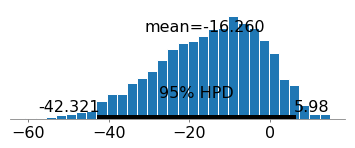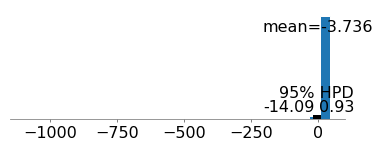Bayesian Logistic Regression in Python using PYMC3In my last post I talked about bayesian linear regression. A fairly straightforward extension of bayesian linear regression is bayesian logistic regression. Actually, it is incredibly simple to do bayesian logistic regression. If you were following the last post that I wrote, the only changes you need to make is changing your prior on y to be a Bernoulli Random Variable, and to ensure that your data is binary. Once you have done that, you are done.

But what if your goal is a little bit deeper than that. One of the really cool things about logistic regression is that you can view it as a latent variable set up. Where you model utility of a decision as a latent variable, and have a decision boundary influenced by this latent variable. This leads, in general terms to the random utility models that underly things like conjoint analysis in the marketing world, and choice experiments in the economics world.

One of the things that always kind of bugged me was that I was modelling this latent variable in a frequentist setting. I thought that it was cool, that you could transform this information into marginal willingness to pay measures. And I spent a fair amount of time in graduate school studying these types of models. The one thing that bugged me though, was that there didn’t seem to be a very good way to estimate the confidence intervals for these willingness to pay metrics. The only way to do it was to use bootstrapping, or one of its variants. It felt kind of clunky to me. It was easy to get point estimates but if you wanted to say that the average willingess to pay was greater than some amount, it felt downright painful.

Enter bayesian models to the rescue.

In this blog post we will see:

• How to estimate a bayesian logistic regression
• Estimate willingness to pay from a bayesian regression
• Estimate the probability that willingness to pay is above a certain amount

Let’s Play With Some Data

Unfortunately, I haven’t done any discrete choice experiments recently. So we’re going to cheat a little bit just to demonstrate the technique. We’ll be using the same data as last time. The dataset that we are going to use is the American Housing Survey: Housing Affordability Data System dataset from 2013. Download it to follow along.

The first thing that we are going to do with this data is prepare it so that it kind of looks like choice experiment data. Now obviously it isn’t but you can imagine that it is similar. We’ll get rid of missing values and code the dependent variable. The way that we are going to do this is to assume that owning a house is the same thing as making a choice for that house. If you rent then you did not “choose” that home. Obviously, there are some serious methodological flaws with this concept of choosing. Again, we’re demonstrating a technique, not trying to publish a paper on the subject.

Here’s the basic code to get the dataset into shape:

import pandas as pd
import pymc3 as pm
import matplotlib.pyplot as plt
import numpy as np
import theano.tensor as t
from scipy.stats import mode
def tinvlogit(x):
return t.exp(x) / (1 + t.exp(x))

df=pd.read_csv('/home/ryan/Documents/thads2013n.txt',sep=',') df=df[df['BURDEN']>0] df=df[df['AGE1']>0] df['OWN']=[1 if obj=='2' else 0 for obj in df['OWNRENT']]

This section of the code should be simple enough. We are just getting the data into python and doing the minor cleaning that we talked about.

Now we need to know how to calculate the WTP from the information that the logistic regression will contain. Essentially, the idea is that if utility exceeds some threshold, then we will see the person owning, otherwise, we’ll see them renting. We model this behavior with a logistic, or sigmoid, transformation. This will give us the probability that we observe ownership given the data.

So it all comes down to the utility. Which we will be modelling as a linear function of the covariates and price. At this point, it makes sense that we will see ownership if we have a non-negative utility. So if utility is modelled like this:$U=\beta_{0}+\beta_{price}Price+\beta_{rooms}Bedrooms$

Then by setting U equalt to zero and solving for price. We get this expression:$Price=-\dfrac{\beta_{0}+\beta_{rooms}Bedrooms}{\beta_{price}}$

And then to get the marginal williness to pay for a bedroom, we find that by taking the derivative with respect to$Bedrooms$. Which results in this function:$mWTP=-\dfrac{\beta_{rooms}}{\beta_{price}}$

And with that we are ready to derive the posterior distribution for our willingness to pay measure. We can do that with the following code:

with pm.Model() as model:
# Define priors
intercept = pm.Normal('Intercept', 0, sd=20)
x_coeff = pm.Normal('x', 0, sd=20)
price_coef = pm.Normal('price', 0, sd=20)

# Define likelihood
likelihood = pm.Bernoulli('y',
pm.math.sigmoid(intercept+x_coeff*df['BEDRMS']+price_coef*df['COSTMED']),
observed=df['OWN'])
WTP=pm.Deterministic('WTP',-x_coeff/price_coef)

# Inference!
trace = pm.sample(3000)

pm.traceplot(trace)

Running this doesn’t seem to be too bad. It only took a few minutes on my older laptop, only about 10ish minutes. So on a relatively new laptop it should run just fine. To view the posterior distributions for the parameters of this model, and for the willingness to pay metric, this code will retrieve them:

pm.traceplot(trace)
plt.show()

Which will give you this plot:Iterestingly, it looks like our WTP metric has a very long tail. And we should believe that there are some really small but positive probability that marginal willingness to pay for another room is very negative. By plotting the posterior for this variable by itself, we can see the high probability density region for this metric, and it is only minorly negative. You can do that with this code:

pm.plot_posterior(trace['WTP'])
plt.show()

And here is the plot where we can see that there is a 95% chance that willingness to pay is between $0.93 per month and -$14.09 per month.We can also find the most probable value for willingness to pay by taking the mode of the posterior distribution which is done using this code:

print(mode(trace['WTP']))

And we find that the most probable WTP is \$13.28. And that’s a basic discrete choice logistic regression in a bayesian framework. Sort of, like I said, there are a lot of methodological problems, and I would never try to publish this as a scientific paper. I was merely demonstrating the technique in python using pymc3. Here is the full code:

#!/usr/bin/env python3
# -*- coding: utf-8 -*-
"""
Created on Tue Jul 11 07:59:54 2017

@author: ryan
"""

import pandas as pd
import pymc3 as pm
import matplotlib.pyplot as plt
import numpy as np
import theano.tensor as t
from scipy.stats import mode
def tinvlogit(x):
return t.exp(x) / (1 + t.exp(x))

df=df[df['COSTMED']>0]
df=df[df['BEDRMS']>0]
df['OWN']=[1 if obj=='2' else 0 for obj in df['OWNRENT']]

with pm.Model() as model:
# Define priors
intercept = pm.Normal('Intercept', 0, sd=20)
x_coeff = pm.Normal('x', 0, sd=20)
price_coef = pm.Normal('price', 0, sd=20)

# Define likelihood
likelihood = pm.Bernoulli('y',
pm.math.sigmoid(intercept+x_coeff*df['BEDRMS']+price_coef*df['COSTMED']),
observed=df['OWN'])
WTP=pm.Deterministic('WTP',-x_coeff/price_coef)

# Inference!
trace = pm.sample(3000)

pm.traceplot(trace)
plt.show()

pm.plot_posterior(trace['WTP'])
plt.show()

print(mode(trace['WTP']))